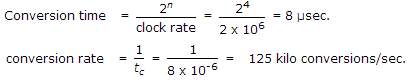# Electronics and Communication Engineering - Digital Electronics

36.

7BF16 = __________ 2

 A. 0111 1011 1110 B. 0111 1011 1111 C. 0111 1011 0111 D. 0111 1011 0011

Explanation:

7BF16 = 7 x 162 + 11 x 161 + 15 x 160 = 1983 in decimal = 0111 1011 1111 in binary.

37.

For the minterm designation Y = ∑ m (1, 3, 5, 7) the complete expression is

 A. Y = A BC + A B C B. Y = A B C + A B C + ABC + A BC C. Y = A B C + A B C + ABC + A BC D. Y = A B C + ABC + A BC + A BC

Explanation:

Decimal number 1 = binary number 001 = A BC Decimal number 7 = binary number 111= ABC, Decimal number 3 = binary number 011= ABC Decimal number 5 = binary number 101= ABC . Hence result.

38.

Zero suppression is not used in actual practice.

 A. True B. False

Explanation:

Zero suppression is commonly used.

39.

A counter type A/D converter contains a 4 bit binary ladder and a counter driven by a 2 MHz clock. Then conversion time

 A. 8 μ sec B. 10 μ sec C. 2 μ sec D. 5 μ sec

Explanation:40.

The hexadecimal number (3E8)16 is equal to decimal number

 A. 1000 B. 982 C. 768 D. 323